# Recherche / Research

Here are my main results:

##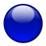Pťnalisťes de l'ťquation des ondes / Penalized Wave Equation

 A. Bachelot-Motet, Une estimation a priori sur les solutions de l'ťquation des ondes pťnalisťe, en dimension 1 d'espace. (in French)
[An a priori estimate for the solutions of the penalized problem: ▢ uε=(1/ε)uε-, in one space variable]
C. R. Acad. Sci. Paris Sťr. I Math. 299 (1984), no. 14, 659-662.

 A. Bachelot-Motet, Une estimation a priori sur les solutions de l'ťquation des ondes pťnalisťe, en dimension 1 et 2 d'espace. (in French)
Proceedings of the 209th EUROMECH Colloquium, 1986, 56-68.

##Rťsonances d'un trou noir de Schwarzschild / Schwarzschild Black-Hole Resonances

 A. Bachelot, A. Motet-Bachelot,
Les pŰles de rťsonance de la mťtrique de Schwarzschild. (in French) [Resonance poles of the Schwarzschild metric]
C. R. Acad. Sci. Paris Sťr. I Math. 316 (1993), no. 8, 795–798.

 A. Bachelot, A. Motet-Bachelot,
Les rťsonances d'un trou noir de Schwarzschild. (in French) [The resonances of a Schwarzschild black hole]
Ann. Inst. H. Poincarť Phys. Thťor. 59 (1993), no. 1, 3–68. Article in PDF

Abstract. This paper is devoted to the theoretical and computational investigations of the scattering frequencies of scalar, electromagnetic, gravitational waves around a spherical Black Hole. We adopt a time dependent approach: construction of wave operators for the hyperbolic Regge-Wheeler equation; asymptotic completeness; outgoing and incoming spectral representations; meromorphic continuation of the Heisenberg matrix; approximation by dumping and cut-off of the potentials and interpretation of the semi group Z (t) in the framework of the Membrane Paradigme. We develop a new procedure for the computation of the resonances by the spectral analysis of the transient scattered wave, based on Prony's algorithm.

 A. Bachelot, A. Motet-Bachelot,
Resonances of Schwarzschild black holes,
Nonlinear hyperbolic problems: theoretical, applied, and computational aspects (Taormina, 1992), 33–40,
Notes Numer. Fluid Mech., 43, Friedr. Vieweg, Braunschweig, 1993.

 A. Motet-Bachelot,
Resonances of the Schwarzschild metric.
Nonlinear evolutionary partial differential equations (Beijing, 1993), 483–487,
AMS/IP Stud. Adv. Math., 3, Amer. Math. Soc., Providence, RI, 1997.

##Equation non-linťaire de Dirac en mťtrique de Schwarzschild  / Nonlinear Dirac Equation in Schwarzschild Metric

 A. Bachelot-Motet,
Nonlinear Dirac fields on the Schwarzschild metric,
Classical Quantum Gravity 15 (1998), no. 7, 1815–1825.

We prove the nonexistence on the Schwarzschild metric of particular stationary states for the nonlinear Dirac equation. In the case where the Schwarzschild metric is restricted to the exterior of a massive star, we investigate numerically the existence of such stationary states with MIT boundary condition.

Here are some stationnary states outside a massive star with the MIT bag condition. Later, our conjecture has been proved by E. Paturel in his paper: A new variational principle for a nonlinear Dirac equation on the Schwarzschild metric, Comm. Math. Phys. 213 (2000), no. 2, 249–266.

`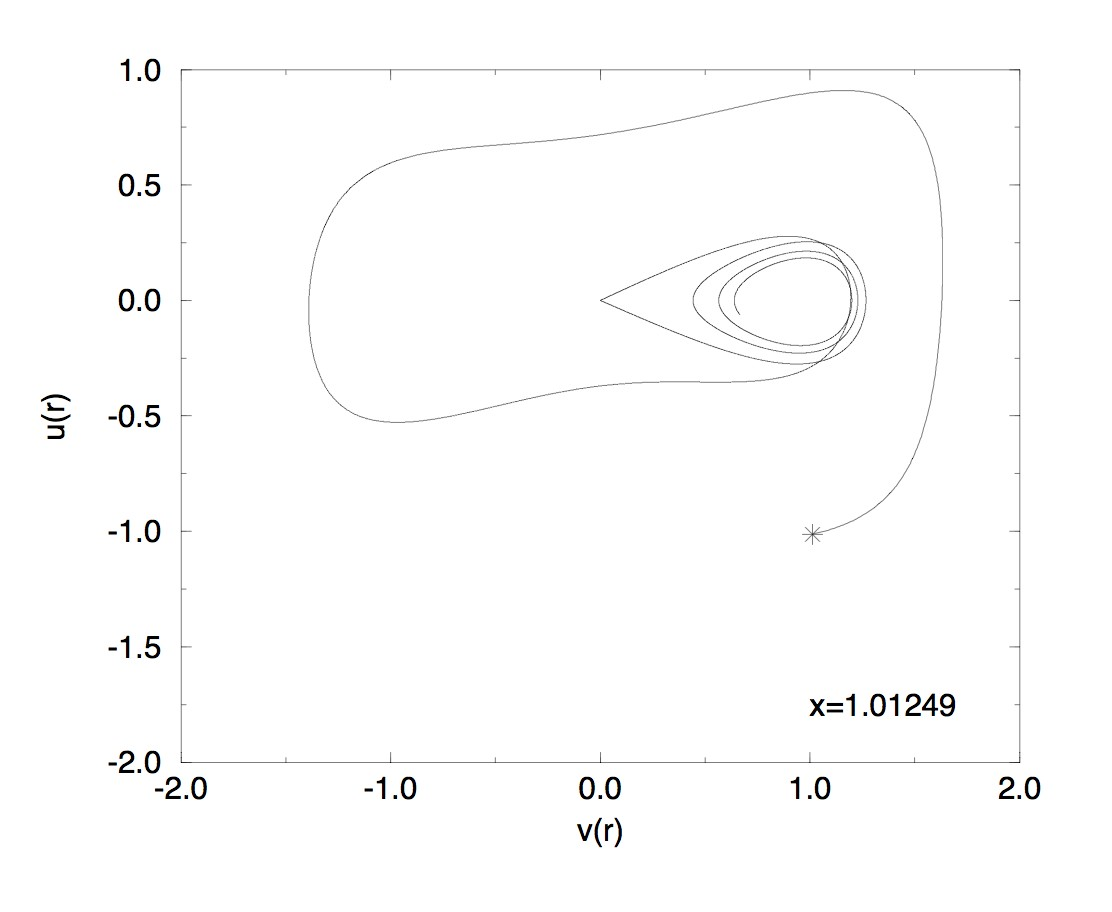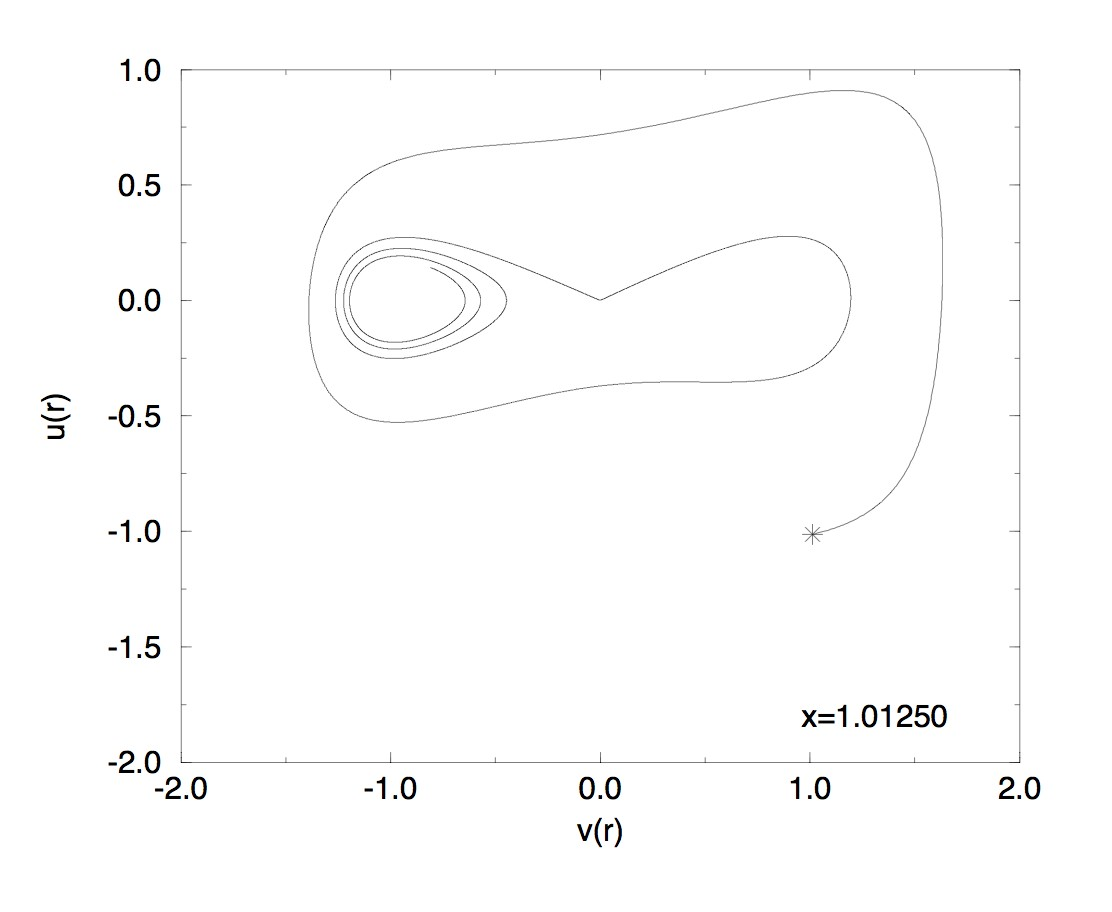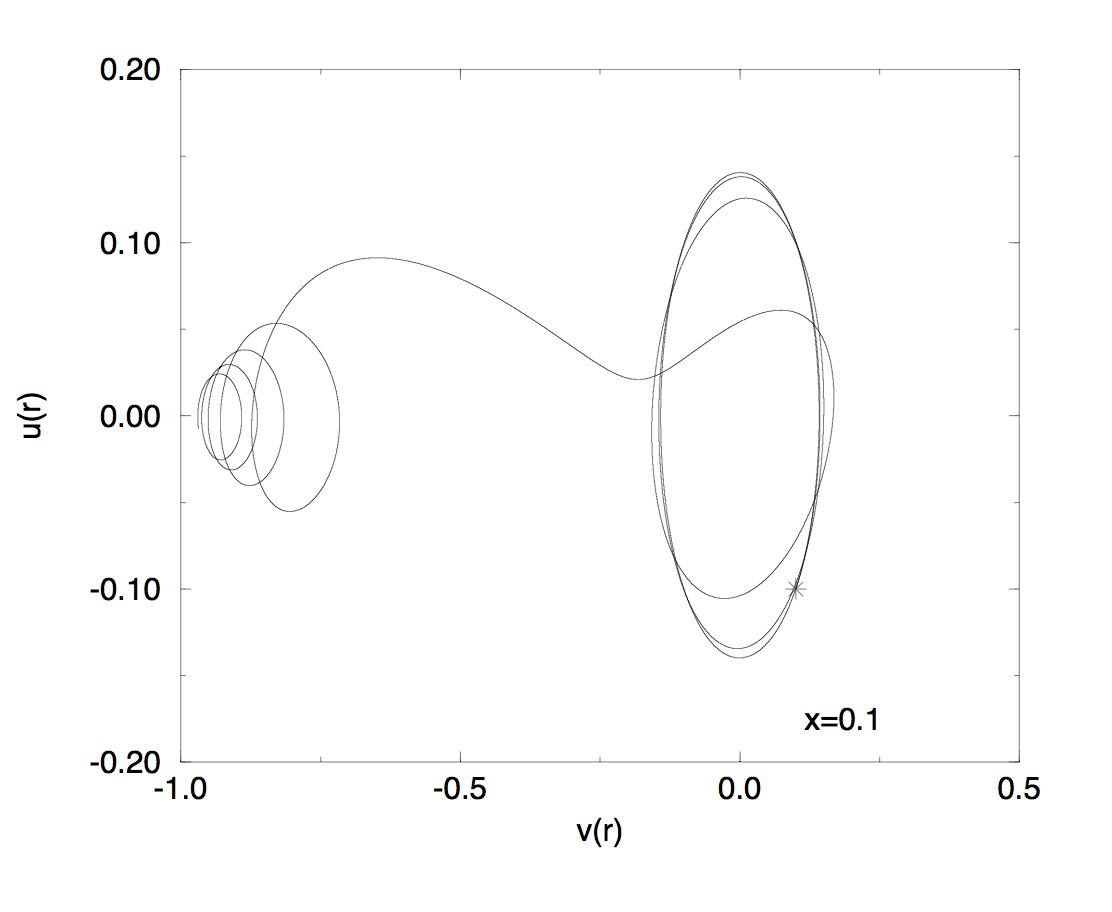`

##Rťsolution numťrique de l'ťquation des ondes sur la surface de genre 2 et de courbure constante ťgale ŗ -1/ Wave computation on a surface of genus 2 and constant curvature -1

 A. Bachelot-Motet, Wave computation on the hyperbolic double doughnut,
J. Comput. Math. 28 (2010), no. 6, 790–806. ARXIV PDF

We compute the waves propagating on the compact surface of constant negative curvature and genus 2 that is a toy model in quantum chaos theory and cosmic topology. We adopt a variational approach using finite elements. We have to implement the action of the fuchsian group by suitable boundary conditions of periodic type. Despite the ergodicity of the dynamics that is quantum weak mixing, the computation is very accurate. A spectral analysis of the transient waves allows to compute the spectrum and the eigenfunctions of the Laplace-Beltrami operator. We test the exponential decay due to a localized dumping satisfying the assumption of geometric control.

The fundamental domain with a mesh, suitable for the hyperbolic metric.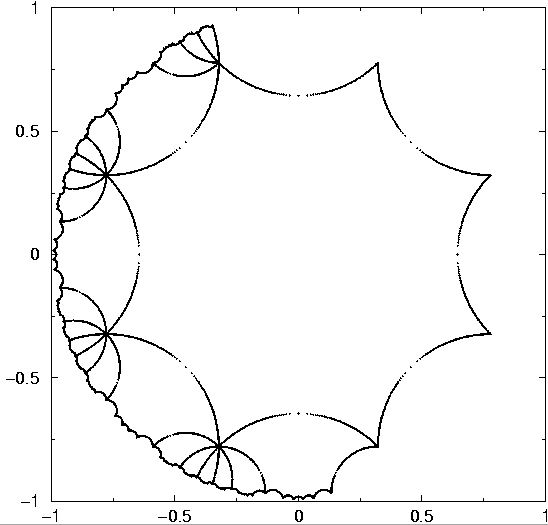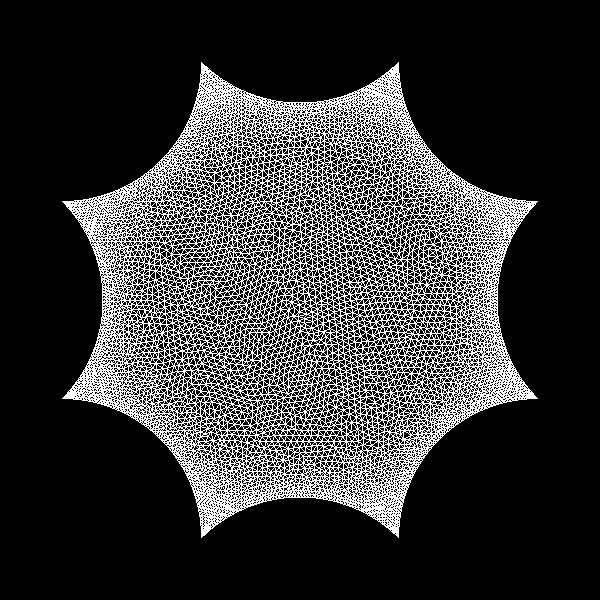Visualization of three solutions:

Centered initial data: the solution  is symetrical

Occurrence of a chaotic behaviour

Just for beauty:

##Rťsolution numťrique de l'ťquation des ondes sur l'espace dodťcaťdrique de Poincarť / Wave Computation on the Poincarť dodecahedral space.

 A. Bachelot-Motet, Wave computation on the Poincarť dodecahedral space,
Classical Quantum Gravity 30 (2013), no. 23, 235010, 31 pp. ARXIV PDF

We compute the waves propagating on a compact 3-manifold of constant positive curvature with a non-trivial topology: the Poincarť dodecahedral space that is a plausible model of multi-connected universe. We transform the Cauchy problem to a mixed problem posed on a fundamental domain determined by the quaternionic calculus. We adopt a variational approach using a space of finite elements that is invariant under the action of the binary icosahedral group. The computation of the transient waves is validated with their spectral analysis by computing a lot of eigenvalues of the Laplace-Beltrami operator.

For some details, see so this page.

##Propagation d'ondes dans des univers dodťcaŤdriques en accťlťration / Waves on accelerating dodecahedral universes.

 A. Bachelot-Motet, A. Bachelot, Waves on accelerating dodecahedral universes,
Classical Quantum Gravity 34 (2017), no. 5, 055010, 39 pp. ARXIV PDF

We investigate the wave propagation on a compact 3-manifold of constant positive curvature with a non trivial topology, the Poincarť dodecahedral space, when the scale factor is exponentially increasing. We prove the existence of a limit state as t tends to infinity and we get its analytic expression. The deep sky is described by this asymptotic profile thanks to the Sachs-Wolfe formula. We transform the Cauchy problem into a mixed problem posed on a fundamental domain determined by the quaternionic calculus. We perform an accurate scheme of computation: we employ a variational method using a space of second order finite elements that is invariant under the action of the binary icosahedral group.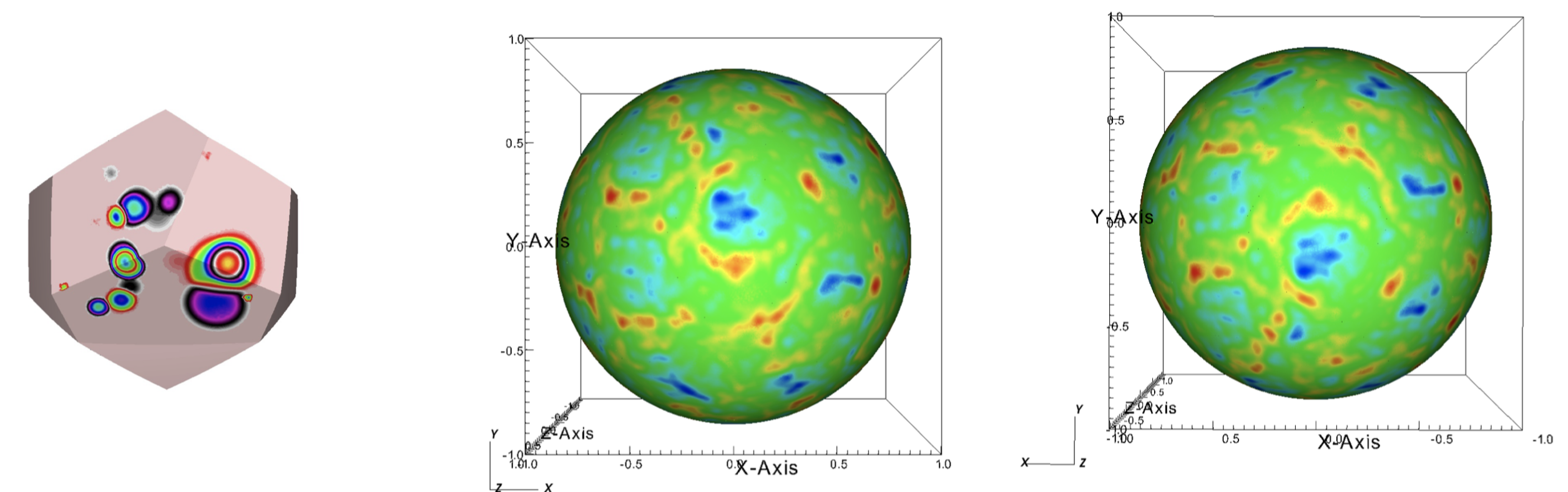An initial random fluctuation and two antipodal views of the deep sky.